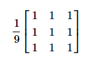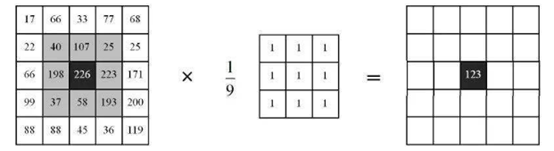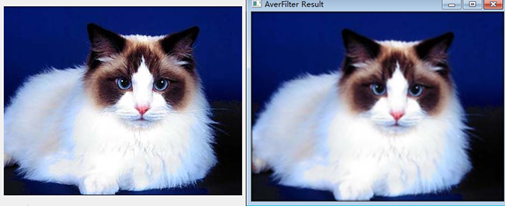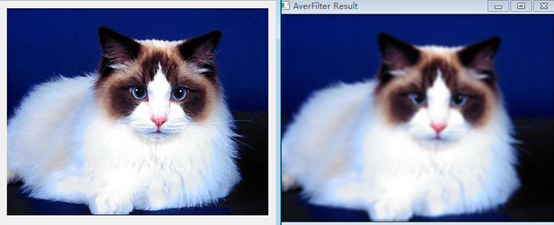# 均值滤波

（1）目的：去除图像上的尖锐噪声，平滑图像。

（2）原理：均值滤波属于线性滤波，它的实现原理是邻域平均法。其中，公式①的Sxy表示中心点在(x,y)处，M表示大小为m×n的滤波器窗口，M=(2m+1)(2n+1)，m和n可以相等。实际上就是用取均值的方式替换原图像中的像素值，即选择一个大小为M模板，该模板由其近邻的若干像素组成，求模板中所有像素的均值，最后填充到输出图像中。g(s , t)表示原始图像， f(x,y)表示均值滤波后得到的图像。

3*3的核示例：（1）判断卷积核是否为偶数，如果是偶数就退出；

（2）边缘处理；

（3）判断图片的通道数，单通道和多通道需要分开处理；

（4）通过公式①计算各点的值；

（5）将各点的值存储到Mat对象中

（6）显示Mat对象，查看均值滤波处理后的结果；

Void AverFilter(Mat& src, Mat& dst, Size wsize)

{

If(卷积核Ksize为偶数)

报异常，退出

边缘处理

If(图片通道数等于1){

单通道的方式求像素点均值

处理结果赋值到输出图像中

}

If(图片通道数大于1){

多通道的方式求像素点均值

处理结果赋值到输出图像中

}

}

void MeanFilater(Mat& src, Mat& dst, Size wsize) {
//判断矩阵的行列数为奇数
if (wsize.height % 2 == 0 || wsize.width % 2 == 0) {
exit(-1);
}
int hh = (wsize.height - 1) / 2;
int hw = (wsize.width - 1) / 2;

//边缘处理

Mat Newsrc;
copyMakeBorder(src, Newsrc, hh, hh, hw, hw, BORDER_REFLECT_101);//以边缘为轴，对称
dst = Mat::zeros(src.size(), src.type());

int sum1 = 0, sum2 = 0, sum3 = 0;
int average1 = 0, average2 = 0, average3 = 0;

for (int i = hh; i < src.rows + hh; i++) {
//Vec3b* src_rows_ptr = Newsrc.ptr<Vec3b>(i);
for (int j = hw; j < src.cols + hw; j++) {
for (int r = i - hh; r <= i + hh; r++) {
Vec3b* new_ptr = Newsrc.ptr<Vec3b>(r);
for (int k = j - hh; k <= j + hh; k++)
{
sum1 += new_ptr[k];
sum2 += new_ptr[k];
sum3 += new_ptr[k] ;
}
}

average1 = sum1 / (wsize.area());
average2 = sum2 / (wsize.area());
average3 = sum3 / (wsize.area());

Vec3b* dst_ptr = dst.ptr<Vec3b>(i - hh);

dst_ptr[j - hw] = average1;
dst_ptr[j - hw] = average2;
dst_ptr[j - hw] = average3;

sum1 = 0, sum2 = 0, sum3 = 0;

average1 = 0, average2 = 0, average3 = 0;
}
}
}posted @ 2021-04-28 10:31  will-z  阅读(797)  评论(0编辑  收藏  举报